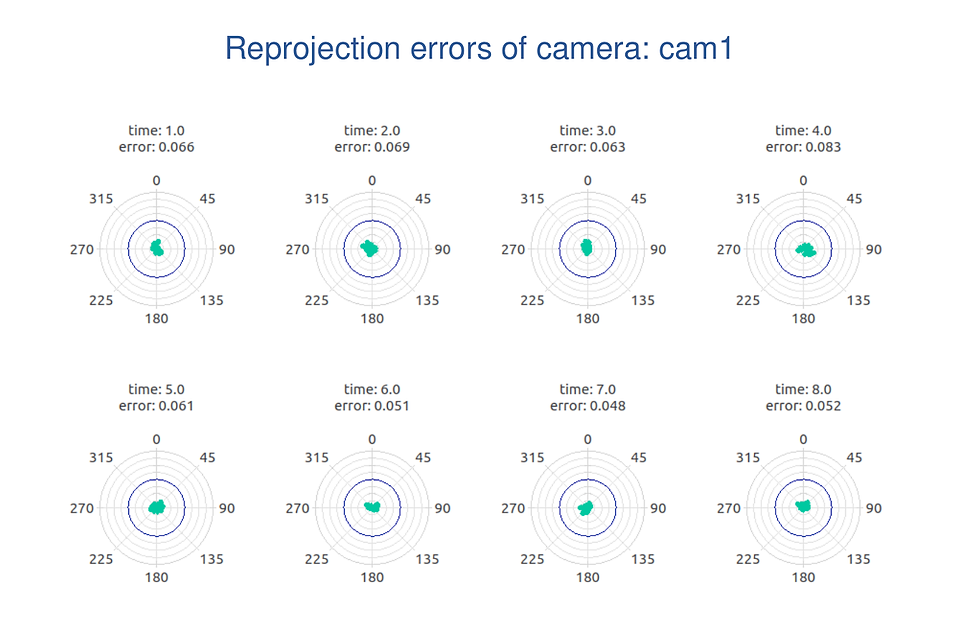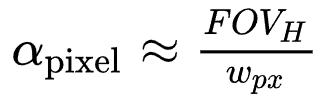top of page
Search

# The Reprojection Error?

The reprojection error measures the distance between the reprojection of a model estimation and its corresponding true projection.

In our case, the model is the intrinsic and extrinsic camera calibration, and the true projections are the observed feature points of the ChArUco or AprilTag board. We measure the reprojection error in pixels on the image plane. Check out the sketch below to get a visual intuition for the reprojection error.Schematic of the reprojection error

The reprojection error is a camera- and setup-independent error metric used to measure the performance of the calibration algorithm (our model) while ignoring external influences.

## Reprojection error and camera calibration

Camera calibration works by measuring a series of feature points whose spatial relation to one another is precisely known. The measured feature points are indicated as blue circles with green center dots in the image below. We find the precise spatial inter-relation of the feature points during board fabrication. The more feature point observations/measurements we have, the better we can model the imaging system – intrinsically and extrinsically.Observed feature points of a ChArUco board encircled in blue.

Once we have modeled (calibrated) the imaging system, we can also conclude the 3D positions of all observed feature points. We generate a set of feature point model predictions when we take the internal 3D model of all feature points and project them back into the scene using the camera and distortion models. The better the model predictions of the feature points and their corresponding observations line up, the lower the reprojection error is.

Expressed differently, we compute the reprojection error as the average L2 norm of point correspondence errors:p_i are the observed feature points on the image plane and q_i are the predicted image plane locations of the 3D feature points when projected onto the image plane and distorted using the lens model parameters from the calibration results.

Tip: check out the camcalib PDF report to inspect the reprojection error of all observations for each camera frame used during calibration. Here is a screenshot of the relevant section in the PDF to save you time searching for it.Reprojection error for each point pi and for each recorded frame drawn in turquoise. The blue circle represents a 0.1pixel reprojection error.

## What does the reprojection error mean to your application?

Recall the pinhole camera model, where every pixel has a unique ray that passes through a tiny hole and sees a minuscule portion of the scene. Essentially, each square pixel observes a tiny pyramid of the scene, and all pyramids originate in the camera's focal point.Sketch of the volume a single pixel observes.

In a first approximation, the pyramid tip angle of all pixelsis estimated as an equal share of the camera's horizontal field of view angle FOV_H over the horizontal pixel count w_px. This means that all pixel measures are convertible to angle measures when you know the sensor geometry and lens parameters (FOV) – in other words when you have a calibrated camera.

Converting the reprojection error e_reproject into an arc-error (in radians) is as simple asIf you know the working distance of your application, you may even go a step further and estimate a length-errorNote that this simple model is increasingly less accurate towards the image borders and for larger FOVs. Our aim here is to convey the basic concept without going into unnecessary detail.

## Example

Let's use our stereo camera use-case as an example to estimate real-world error expectations from the reprojection error.

We achieved a reprojection error of 0.053 pixels in the use case. We know from the intrinsic calibration results that the sensor is 1280 pixels wide and that the horizontal focal length is fx=2282.3921 pixels. Withwe conclude that the camera system has a horizontal FOV of 31.3°. Usingwe find the approximate pixel angle to be 0.024°. Therefore, our reprojection error of 0.053 pixels works out to be 22.6µrad or 0.0013°.

If we assume a working distance of 2 meters, our length error is 45.2µm.

## In conclusion

You should now have a good understanding of the concepts behind the reprojection error and should easily be able to estimate the impact it has on your application.

Please reach out to us if you have further questions or need support in accurately estimating the reprojection error distribution for your specific application. We are happy to help.